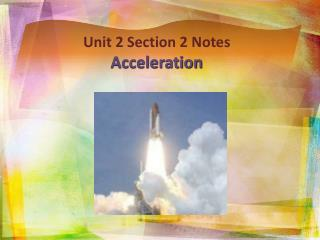DownloadDownload PresentationUnit 2 Section 2 Notes Acceleration

Unit 2 Section 2 Notes Acceleration

Télécharger la présentationUnit 2 Section 2 Notes Acceleration

- - - - - - - - - - - - - - - - - - - - - - - - - - - E N D - - - - - - - - - - - - - - - - - - - - - - - - - - -
Presentation Transcript

1. Unit 2Section 2 Notes Acceleration

2. Acceleration • Acceleration: rate of change in velocity • An object accelerates if: • It’s speed changes: if an object is speeding up or slowing down, its acceleration is changing • It’s direction changes: if an object is traveling at the same speed but changes direction • Both speed and direction change • Acceleration is a vector quantity: includes both magnitude and direction.

3. Acceleration • An object is accelerating even it its speed stays the same and only its direction changes. Why? • Acceleration is defined as the rate at which velocity changes with time; velocity includes both speed and direction, so an object accelerates if its speed, direction, or both change. • Therefore, you can constantly accelerate while never speeding up or slowing down!

4. Centripetal Acceleration • Centripetal Acceleration: acceleration that occurs in a circular motion. • If you move at a constant speed in a circle, even though your speed doesn’t change, your direction does; therefore, you are accelerating. • Examples: • The moon accelerates around the earth • Riding on a Ferris wheel • Blades on a windmill • Roller coaster

5. To find the acceleration of an object in a straight line, you need to measure the object’s velocity at different times. To mathematically find acceleration, take the change in an object’s velocity divided by time. or Calculating Acceleration

6. Acceleration Values • What do acceleration values tell you? • If acceleration is small, velocity is increasing very gradually. • If acceleration is large, velocity is increasing more rapidly. • Positive acceleration: an object’s velocity is increasing; object is speeding up • Negative acceleration: an object’s velocity is decreasing; object is slowing down

7. Acceleration Values • In science, acceleration describes any change in velocity; not just speeding up • When you slow down, you have a negative acceleration because it is opposite the direction of motion.

8. Graphing Accelerated Motion • When graphing acceleration: • Independent variable, which is time, is on the x-axis • Dependent variable, which is speed/velocity, is on the y-axis

9. Graphing Accelerated Motion • A straight line on a speed vs. time graph means the speed changes by the same amount over equal time intervals. This is called constant acceleration. • The slope of a straight line on a speed vs. time graph is equal to acceleration. • Line with a positive slope: object is speeding up • Line with a negative slope: object is slowing down • A curved line on a distance vs. time graph means the object is accelerating.

10. Acceleration Sample Problems • Natalie accelerates her skateboard along a straight path from rest to 4.5 m/s in 2.5 seconds. Find her average acceleration.

11. Acceleration Sample Problems • Find the average acceleration of a northbound subway train that slows down from 12 m/s to 9.6 m/s in 8 s.

12. http://www.physicsclassroom.com/Class/1DKin/U1L1e.cfm